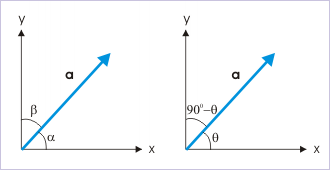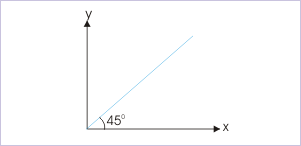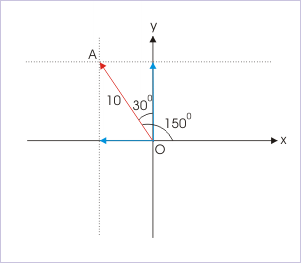# 1.6 Components of a vector  (Page 2/4)

 Page 3 / 4

## Planar components of a vector

The planar components of a vector lies in the plane of vector. Since there are two perpendicular axes involved with a plane, a vector is resolved in two components which lie in the same plane as that of vector. Clearly, a vector is composed of components in only two directions :

$\begin{array}{l}\mathbf{a}=a\mathrm{cos}\alpha \mathbf{i}+a\mathrm{cos}\beta \mathbf{j}\end{array}$

From the figure depicting a planar coordinate, it is clear that angle “β” is compliment of angle “α”. If α = θ, thenThe direction of a planar vector with respect to rectangular axes can be described by a single angle.

$\begin{array}{l}\alpha =\theta \phantom{\rule{4pt}{0ex}}\mathrm{and}\phantom{\rule{4pt}{0ex}}\beta =90°-\theta \end{array}$

Putting in the expression for the vector,

$\begin{array}{l}\mathbf{a}={a}_{x}\mathbf{i}+{a}_{y}\mathbf{j}\\ \mathbf{a}=a\mathrm{cos}\theta \mathbf{i}+a\mathrm{cos}\left(90°-\theta \right)\mathbf{j}\\ \mathbf{a}=a\mathrm{cos}\theta \mathbf{i}+a\mathrm{sin}\theta \mathbf{j}\end{array}$

From graphical representation, the tangent of the angle that vector makes with x-axis is :

$\begin{array}{l}\mathrm{tan}\alpha =\mathrm{tan}\theta =\frac{a\mathrm{sin}\theta }{a\mathrm{cos}\theta }=\frac{{a}_{y}}{{a}_{x}}\end{array}$

Similarly, the tangent of the angle that vector makes with y-axis is :

$\begin{array}{l}\mathrm{tan}\beta =\mathrm{tan}\left(90°-\theta \right)=\mathrm{cot}\theta =\frac{a\mathrm{cos}\theta }{a\mathrm{sin}\theta }=\frac{{a}_{x}}{{a}_{y}}\end{array}$

Problem : Find the unit vector in the direction of a bisector of the angle between a pair of coordinate axes.

Solution : The unit vector along the direction of a bisector lies in the plane formed by two coordinates. The bisector makes an angle of 45° with either of the axes. Hence, required unit vector is :Unit vector along the bisector.

$\begin{array}{c}\mathbf{n}=\mathrm{cos}\theta \mathbf{i}+\mathrm{sin}\theta \mathbf{j}=\mathrm{cos}45°\mathbf{i}+\mathrm{sin}45°\mathbf{j}\\ ⇒\mathbf{n}=\frac{1}{\surd 2}\phantom{\rule{2pt}{0ex}}x\phantom{\rule{2pt}{0ex}}\left(\mathbf{i}+\mathbf{j}\right)\end{array}$

Note : We may check that the magnitude of the unit vector is indeed 1.

$\begin{array}{c}|\mathbf{n}|=n=\surd \left\{{\left(\frac{1}{\surd 2}\right)}^{2}+{\left(\frac{1}{\surd 2}\right)}^{2}\right\}=\surd 1=1\end{array}$

## Representation of a vector in component form

The angle involved in determination of components plays important role. There is a bit of ambiguity about angle being used. Actually, there are two ways to write a vector in component form. We shall illustrate these two methods with an illustration. Let us consider an example. Here, we consider a vector OA having magnitude of 10 units. The vector makes 150° and 30° angle with x and y axes as shown in the figure.Component of a vector

Using definition of components, the vector OA is represented as :

$\mathbf{OA}=10\mathrm{cos}{150}^{0}\mathbf{i}+10\mathrm{cos}{30}^{0}\mathbf{j}$ $⇒\mathbf{OA}=10X-\frac{1}{2}\mathbf{i}+10X\frac{\sqrt{3}}{2}\mathbf{j}$ $⇒\mathbf{OA}=-5\mathbf{i}+5\sqrt{3}\mathbf{j}$

Note that we use angles that vector makes with the axes to determine scalar components. We obtain corresponding component vectors by multiplying scalar components with respective unit vectors of the axes involved. We can, however, use another method in which we only consider acute angle – irrespective of directions of axes involved. Here, vector OA makes acute angle of 60° with x-axis. While representing vector, we put a negative sign if the component is opposite to the positive directions of axes. We can easily determine this by observing projection of vector on the axes. Following this :

$\mathbf{OA}=-\mathrm{cos}{60}^{0}\mathbf{i}+10\mathrm{sin}{60}^{0}\mathbf{j}$ $⇒\mathbf{OA}=-5\mathbf{i}+5\sqrt{3}\mathbf{j}$

Note that we put a negative sign before component along x-direction as projection of vector on x-axis is in opposite direction with respect to positive direction of x-axis. The y - projection, however, is in the positive direction of y-axis. As such, we do not need to put a negative sign before the component. Generally, people prefer second method as trigonometric functions are positive in first quadrant. We are not worried about the sign of trigonometric function at all.

#### Questions & Answers

List the application of projectile
Luther Reply
How can we take advantage of our knowledge about motion?
Kenneth Reply
pls explain what is dimension of 1in length and -1 in time ,what's is there difference between them
Mercy Reply
what are scalars
Abdhool Reply
show that 1w= 10^7ergs^-1
Lawrence Reply
what's lamin's theorems and it's mathematics representative
Yusuf Reply
if the wavelength is double,what is the frequency of the wave
Ekanem Reply
What are the system of units
Jonah Reply
A stone propelled from a catapult with a speed of 50ms-1 attains a height of 100m. Calculate the time of flight, calculate the angle of projection, calculate the range attained
Samson Reply
58asagravitasnal firce
Amar
water boil at 100 and why
isaac Reply
what is upper limit of speed
Riya Reply
what temperature is 0 k
Riya
0k is the lower limit of the themordynamic scale which is equalt to -273 In celcius scale
Mustapha
How MKS system is the subset of SI system?
Clash Reply
which colour has the shortest wavelength in the white light spectrum
Mustapha Reply
how do we add
Jennifer Reply
if x=a-b, a=5.8cm b=3.22 cm find percentage error in x
Abhyanshu Reply
x=5.8-3.22 x=2.58
sajjad

### Read also:

#### Get Jobilize Job Search Mobile App in your pocket Now!

Source:  OpenStax, Physics for k-12. OpenStax CNX. Sep 07, 2009 Download for free at http://cnx.org/content/col10322/1.175
Google Play and the Google Play logo are trademarks of Google Inc.

Notification Switch

Would you like to follow the 'Physics for k-12' conversation and receive update notifications?By OpenStaxBy OpenStaxBy Jessica CollettBy Anh DaoBy Robert MorrisBy Dan ArielyBy Yasser IbrahimBy OpenStaxBy OpenStaxBy OpenStax